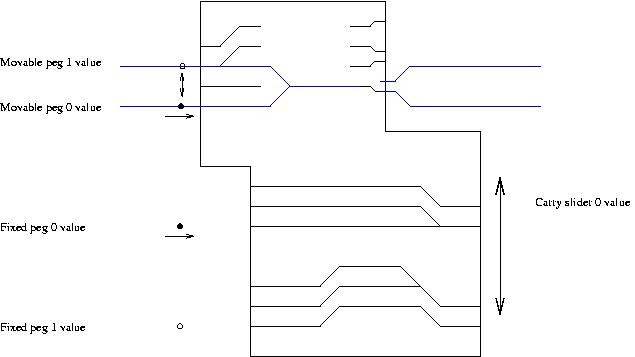# Some thoughts and speculations on Danny Hillis's mechanical binary adder

There are all kinds of wonderful mechanical devices that represent digital operations, ranging from puzzles such as the Chinese Rings to the Babbage differential analyzer. The Hillis mechanical binary adder is in this tradition.

In Spring 2000 I visited the Long Now Foundation offices in the Presidio in San Francisco. Alexander and Camille graciously welcomed a couple of friends and me.

We saw a prototype of the Clock of the Long Now, and Alexander and Camille gave us a guided tour. They explained several fascinating aspects of the workings of the clock.

Based on my recollections of the device and Alexander's comments, I came up with some ideas about the design and function of the binary adder.

I have come up with a version of the binary adder that I think works, but is not as clever as what I remember of Danny's design. (By way of analogy, it's as though Danny came up with a way to solve Rubik's Cube in a maximum of 23 moves, and I can't remember how he did it, but I came up with a solution that takes 33 moves.) (January 28, 2005: I found the patent describing Danny's implementation. I have added a brief description of his solution in an appendix at the bottom of this web page. As predicted, it is indeed clean and elegant, and simpler than mine.)

Although not as clever, minimal, and beautiful as Danny's design, my simpler reconstruction might have some pedagogical interest. This note describes this reconstruction of the operation of the Hillis mechanical binary adder.

One intersting aspect of the design described here is that it is functionally complete (a property shared, for instance, by the NAND gate). This is because it implements a general "NOT" or negation boolean operation in addition to AND and OR.

Any and all mistakes, errors, gaffes, etc. in this note are mine. Any and all credit and thanks go to the Long Now Foundation and Danny Hillis for creating such a beautiful, elegant, and deep vision.

The basic idea of the Hillis binary adder is that it is a wheel that goes around, and after N rotations it engages another wheel and advances it. In this sense the binary adder is like a pair of gears with an N-to-1 gear ratio. (On the Long Now clock, the other wheel only advances 60 degrees each time it is advanced, so the gear ratio is actually 6*N-to-1.)

The way the adder works is that each rotation of the wheel adds a constant value to an accumulator. When the accumulator overflows, the other wheel is engaged and advances.

Through an external gear train, instances of the binary adder control the movement of various dials on the face of the Clock of the Long Now.

The interesting thing about the binary adder is that it is very easy to change its gear ratio. There is a set of 27 pegs, and each of these pegs can be put in one of two positions. Interpreting the positions as the binary digits 0 and 1, there are 2^27 (134,217,728) distinct gear ratios that can be achieved. To change the gear ratio, just re-position the pegs.

The re-positioning could be done manually if some new calendar system were devised and it were desired to change the rate of rotation of the dials on the face of the clock.

Alternatively, the re-positioning of the pegs to change the gear ratio can be done automatically by a mechanism in the clock itself. So, one could imagine one instance of the binary adder being used for multiple purposes within a clock that require different gear ratios.

The binary adder is a mechanical implementation of the following code fragment:

```int old_value, new_value;

/* 0 <= old_value < MAX */
while (true) {

/* 0 <= CONSTANT < MAX */

new_value = (old_value + CONSTANT) % MAX;

if (new_value < old_value) {
/* arithmetic overflow occurred */
}

old_value = new_value;
}
```

The mechanical binary adder I will describe is a straightforward implementation of binary addition with carry propagation. Say we wish to add 7 and 5. In binary, this is the following:

```   1  1  1
+ 1  0  1
---------
```

We start at the rightmost column, add the two numbers together, represent the result in binary, and propagate the carry:

1 + 1 = 10 (2 in binary), so we have a first-column result of 0 and a carry of 1.

Including the carry of one from the first column and adding up the second column from the right:

1 + 0 + 0 = 10 (2 in binary), so the second-column result is 0 and a carry of 1.

The complete sum is

```   1  1
1  1  1
+ 1  0  1
---------
1  1  0  0
```

In addition to the conventional representation given above, there are many other ways to represent the integers in binary. Depending on the operations that need to be implemented and the mechanisms available to do the implementation, one representation may be more convenient than another. While the implementation of the Hillis binary adder described here uses the conventional representation of integers in binary and the standard method of addition, it might be possible to improve or simplify the design with an alternate representation.

As a classic example, if the only operations required are to add 1 or subtract 1, the Gray codes can be used to create clever mechanical implementations of binary arithmetic. In a Gray code, to add or subtract 1, you only have to change a single bit.

Here is a description of a basic mechanism that we will use in this note.

The first part of the mechanism is a rectangular slider that is surrounded by four stationary wheels, and moves up and down inside the wheels, as shown in Figure 1. The slider represents the value of the carry bit during binary addition; the up position represents 1, and the down position represents 0.

Figure 1: The vertical position of the slider represents the carry bit.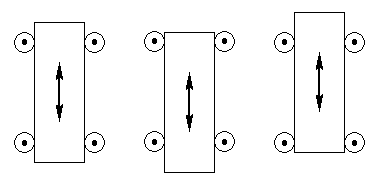The carry slider is borne on a trolley apparatus that moves to the right along a pair of tracks, as shown in Figure 2.

Figure 2: The trolley apparatus moves along to the right, reading the inputs and doing the addition.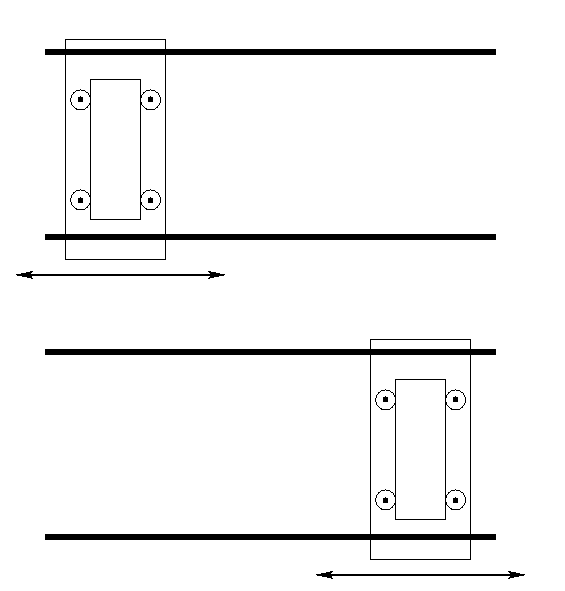The trolley passes over two sets of pegs as it moves to the right. (These will be shown in subsequent figures.) The pegs represent the two binary numbers to be added. The lower set of pegs are in fixed positions. Each of these pegs can be in one of two holes, an upper hole representing a 1, or a lower hole representing a 0. The upper set of pegs can change their positions. Each peg is on an arm that can rotate. If the arm is rotated downward, the peg represents a 0. Conversely, if the arm is rotated upward, the peg represents a 1. (This design will be discussed more thoroughly, with the aid of some figures, below.)

As the trolley moves along to the right, it re-positions the upper pegs as it passes over them. The upper set of pegs together represent the accumulator.

We will build the binary adder in several steps. The first step is to construct an OR gate, shown in Figure 3. The trolley moves left to right over the pegs. In this figure, all of the pegs have been placed in the lower hole, representing zero or false, and the trolley itself starts out in the down position, also representing zero or false. The trolley will move all the way to the right and stay in the low position. The position of the carry slider at the end indicates the result of the operation, namely false.

Figure 3: Implementation of an OR operation.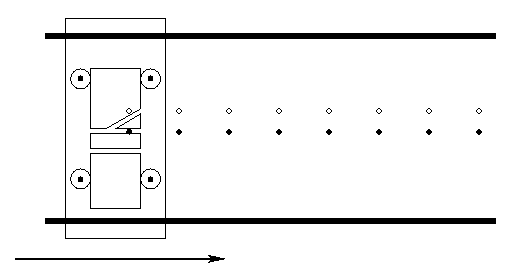In Figure 4, one of the pegs is in the true position, so the carry slider will move up when it encounters that peg. After that it will stay in the true position, and so the result at the end is true.

Figure 4: The OR gate, with a bit set to true, causing the result to be true.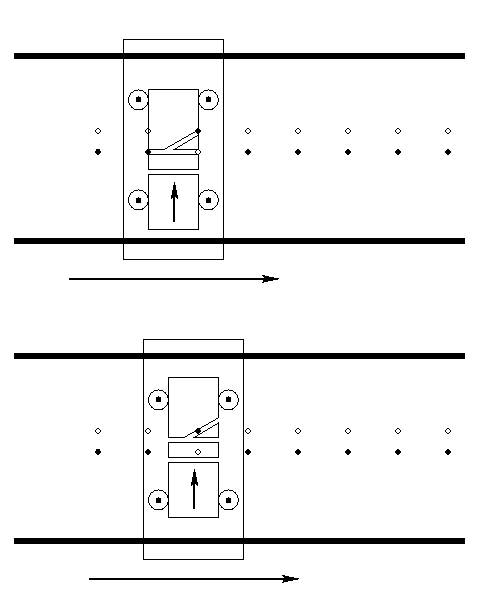In Figure 5, an AND gate is shown. If the carry slider starts off in the false (low) position, or if any of the pegs is in the low position, then the carry slider will end in the low position when it gets all the way to the right. On the other hand, if it starts high and all of the pegs are in the up position, the carry slider will end in the up position at the right.

Figure 5: Implementation of AND operation.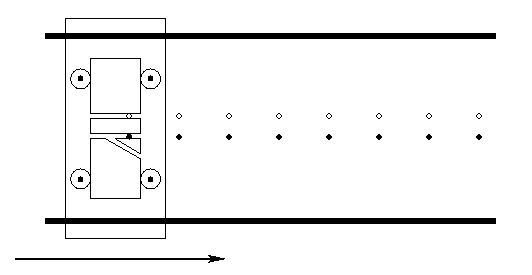In addition to our implementation of AND and OR described above, we will also need to implement a NOT operation, but that discussion will be defered for the moment.

We now examine the implementation of the carry bit. The following binary table is what we have to implement:

```Upper  Carry  Lower  Carry
Peg   Input   Peg   output
0      0      0      0
0      0      1      0
0      1      0      0
0      1      1      1

1      0      0      0
1      0      1      1
1      1      0      1
1      1      1      1
```

Note that if the upper peg is 0, the carry output is an AND operation. If the upper peg is 1, the carry output is an OR operation. This observation will guide our implementation. We will combine the AND and OR gates above to implement the carry bit.

Figure 6 shows the carry slider and two pegs. (As the carvings in the carry slider become more complicated, we adopt a more stylized presentation of the carry slider, omitting the trolley and its track, and representing paths through the carry slider with lines rather than little tunnels.)

The upper peg has two choices for which hole to occupy (it occupies its upper hole in the figure), as does the lower peg. Also, the carry slider can be high or low. In Figure 6 the carry slider is shown in its high position, representing an input value of 1. After passing to the right over the (fixed) pegs, the carry slider will remain in the high position. This corresponds to the last row of the table above.

Figure 6: Implementation of the carry operation.The idea of the carry slider is that the upper peg goes into a hole of the carry slider first. The upper peg then moves the carry slider vertically and chooses which holes will line up with the bottom pegs. The bottom three lines of the carry slider implement the OR operation from above, and the second, third, and fourth lines from the bottom represent the AND operation from above. In Figure 7, the top peg causes the carry slider to move down as it slides along to the right, causing the lower peg to enter the second hole on the lower set of four holes in the slider. But, this is a zero input to an AND gate, so the slider then moves back down. In a slightly round-about way, we have implemented the first row of the carry table.

Figure 7: The carry operation, with inputs 0, 0, 0.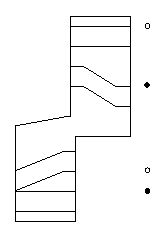One final interesting example is given in Figure 8. The carry slider starts off in the low position, but both pegs are in the respective high positions, and so the carry slider ends up in the high position, implementing row 6 of the table.

Figure 8: The carry operation, with inputs 1, 0, 1.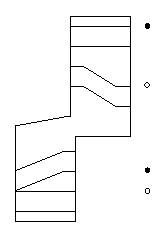My incomplete recollection of Danny Hillis's design indicates that he came up with a superior solution to the above problem. I think his carry slider had only six holes for pegs to enter, rather than the eight of the my design. He somehow managed to use an alternate representation of binary arithmetic, or found some other way to combine paths.

We will now modify the upper peg so that it is variable. That is, the carry slider mechanism can re-position this peg automatically. Figure 9 is an illustration of the upper peg. The two possible positions of the peg are shown with small light and dark circles. The peg in Figure 9 is in the upper position. It is at the end of an arm that pivots. The peg is held stationary in Figure 9 because it is trapped in a chute.

Figure 9: The peg is on an arm that rotates, so that it can be in the upper or lower position.The chute apparatus moves to the right along with the carry slider. Figure 10 shows the chute having moved, and at that point the peg is no longer trapped; it is free to rotate.

Figure 10: The chute apparatus has advanced to the right, and the peg is free to rotate between the upper and lower positions.Figure 11 shows the peg having been rotated down a bit more than halfway, and the chute has advanced. At this point, the peg is trapped in the lower chute, and as the chute apparatus advances farther to the right the peg will be guided down into the lower chute.

Figure 11: The chute apparatus has advanced further to the right, capturing the peg in the lower chute.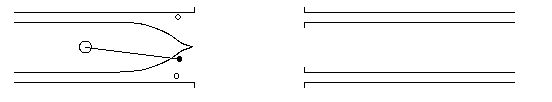Having implemented the carry operation, we must now implement the updating of the accumulator bit, at which point we are done.

The following binary table is what we have to implement for the accumulator. In addition to the inputs and the output, I have added a useful additional column, namely the hole that the lower peg enters as the carry slider moves to the right. This is most easily checked by referring back to Figure 6, the carry slider.

```Upper  Carry  Lower  Upper Peg  Hole Lower
Peg   Input   Peg    output    Peg Enters
0      0      0      0            l1
0      0      1      1            l0
0      1      0      1            l2
0      1      1      0            l1

1      0      0      1            l2
1      0      1      0            l1
1      1      0      0            l3
1      1      1      1            l2
```

The implementation of the accumulator output will be based on an implementation of constant functions. (The inputs to the constant functions are the values of the lower fixed peg, i.e., whether the position of the lower fixed peg encodes 0 or 1.)

Interestingly (and fortunately), it turns out that the output position of the accumulator bit depends solely on which of the four holes in the bottom of the carry slider the lower peg enters, and not on the position of the lower peg or the initial position of the slider. (All entries for l1 in the above table have the same upper peg output, for instance.) So, we simply have to implement four constant functions, one for each of the four lower holes in the carry slider.

Figure 12 gives the full implementation. To make things a little clearer, Figure 13 gives the carry slider and the pegs, and omits the chute apparatus.

In order to reduce the number of line crossings in the paths for the lower pins, it is convenient to switch the interpretations of the positions of the moving pin, so that the `up' position represents 0 and the `down' position represents 1. This includes switching the pairs of parallel paths for the moving pin. After the paths for the moving pin have re-positioned the carry slider to guide the lower fixed pins into their required slots, the four paths for the moving pin feed into a single path. The lower fixed pins then implement their respective required constant functions, guiding the slider up or down as necessary. The carry slider may be in any of three vertical positions, but it is easy to ensure that no matter which of these positions it is in, the single path will dump the moving pin into the correct upper or lower chute so that the moving pin is left in the required 0 or 1 output state.

Figure 12: The entire mechanism, including manipulation of the carry bit and the accumulator bit, but with line crossing.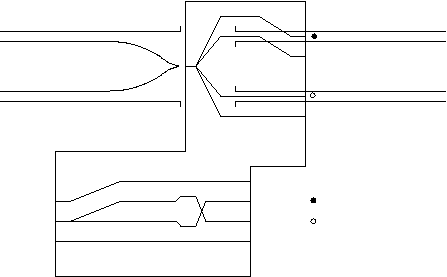As with our first implementation of the carry bit, the first thing that happens is that the upper peg guides the carry slider up or down, and chooses which set of holes the lower peg can enter. The lower peg then implements a constant function and guides the upper peg above or below the point of the chute apparatus, depending on which hole the lower peg entered.

After having guided the upper peg to whichever chute it belongs in, the lower peg moves the carry slider vertically to implement the required output value of the carry bit.

Figure 13: The the carry slider and pins, with line crossing.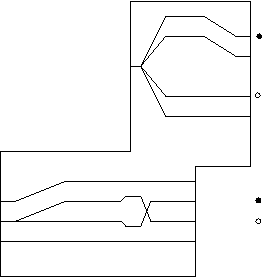A problem with the above idea is that the second and third lines for the fixed pins cross over each other. At the point of cross-over the machine is in an ambiguous state, and the carry slider could move either up or down (or wedge). We address this problem by considering the implementation of a cross-over operation.

We will present two implementations of cross-over. The first implementation is the more conceptually straightforward of the two, and serves as a useful starter exercise. It will not work as a solution to our problem, however, so a second, more complex implementation of cross-over will be given that does suffice for our needs.

The first implementation is given in Figure 14. The upper (fixed) peg can be in one of the two positions shown. We have added a lower 'guide' peg, which is also fixed. As above, the apparatus with the lines moves to the right, and it can move up and down. If the upper peg is in the upper of its two possible positions, the apparatus will be guided to move up, and the peg will exit out of the lower of the two holes at the left of the top part of the apparatus. Conversely for the case where the upper peg starts off in the lower of its two possible positions. The idea is that the upper peg will guide the apparatus up or down a small amount, and put the guide peg into one of its two possible tracks. The guide peg will then follow the selected track, and control the vertical position of the apparatus. It will guide the apparatus across the zone of ambiguity for the upper peg where the two upper lines cross.

Figure 14: A cross-over circuit.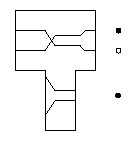If our real carry slider were always in the middle of its three possible vertical positions when the lower peg entered one of the lower holes, then the above cross-over technique would suffice. Unfortunately, that is not the case. In terms of Figure 14, the apparatus can be initially positioned in any of three vertical positions, so that the upper peg can go into either of the two holes, no matter which position it is in. So, we have to resort to a more complicated mechanism to implement the required cross-over.

Figure 15 illustrates the situation we have to solve. The carry slider can be in any of thee possible vertical positions. If the peg is in its top position, it can go into any of the top three paths in the carry slider. If the peg is in its bottom position, it can go into any of the three lower paths in the carry slider. So, there are six possible combinations of peg going into path. Because of symmetry, we only have to consider the top peg. If the slider starts off in the lowest position, the peg enters the top path, and no crossover happens. However, if the top peg enters the second or third path, a crossover is required.

Figure 15: The general cross-over problem; the carry slider can start in any of three vertical positions.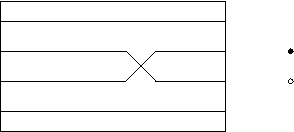To solve the problem, we will attach the guide peg to a movable arm and allow it to move vertically, in a manner similar to the accumulator peg above.

As an aid to intuition, it is useful to change reference frames, and envision the pegs to be moving to the left together. The carry slider is now stationary horizontally, but it can still move up or down. In addition, there is a stationary underlying level under the carry slider that we can put grooves in to hold and control our now movable guide peg.

In the first solution to the crossover problem, we had the guide peg move through tracks in the carry slider. To solve our more general problem, we will have the guide peg move at times through tracks in the underlying level, at other times through tracks in the carry slider, and at yet other times through tracks in both.

We place three horizontal lines in the carry slider, one for each of the possible initial vertical positions of the carry slider. The guide peg enters one of these three lines. Then, the upper peg follows whichever of the two tracks it enters. It moves the carry slider up or down a small amount, carrying the (movable) guide peg with it. The guide peg is put into one of the tracks in the underlying level. As the guide peg moves further to the left, it hits an incline in the underlying level, either up or down, and the guide peg follows that path. It transfers that vertical motion to the carry slider, moving the carry slider vertically with it. As in the previous implementation of cross-over, the guide peg guides the upper (fixed) peg across the ambiguous region of the cross-over point.

Figure 16 shows the carry slider (in black) and the underlying level (in blue), superimposed on each other in their correct positions. This view causes some of the lines to overlap, so in Figure 17 the carry slider and the underlying level are vertically displaced to show all of the lines in the two of them.

(The top part of the carry slider is wide to enable a previous set of pegs (not shown in the diagram) to maintain the vertical position of the carry slider on the left as the next set of pegs enter it from the right.)

Figure 16: Solution to the general crossover problem, with carry slider and underlying level superimposed in their correct positions.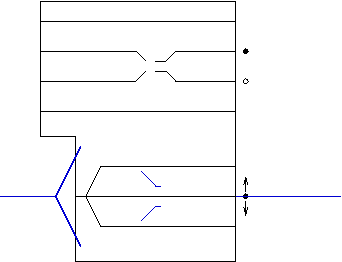Figure 17: Solution to the general crossover problem, with carry slider and underlying level offset to avoid overlaps and show detail of grooves in each.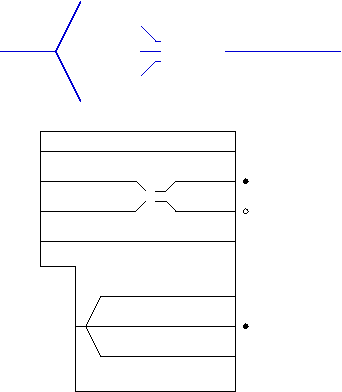Finally, we can put together all of the pieces for the general solution to the problem. For the crossover, we need a movable guide peg. As it turns out, it is possible to use our accumulator peg for this purpose! Going in, the accumulator peg contains state that must be read and preserved. However, that state is transferred to the groove into which the lower peg enters the carry slider. After the transfer of state, we no longer need to use the accumulator peg to remember input state, and we are free to use it to assist in the crossover problem on the lower peg.

Figure 18 shows the carry slider and underlying level, again superimposed in their correct positions. Figure 19 shows the carry slider and the underlying level vertically offset to avoid overlaps and show detail of the grooves in each.

Figure 18: Solution to the general crossover problem, with carry slider and underlying level superimposed in their correct positions.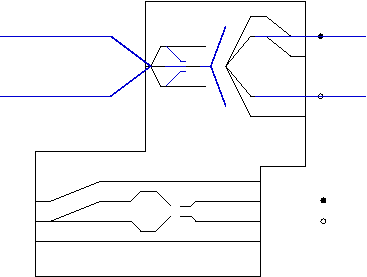Figure 19: Solution to the general crossover problem, with carry slider and underlying level offset to avoid overlaps and show detail of grooves in each.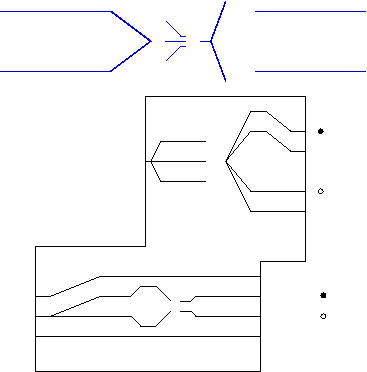An observation about using the binary adder to add multiple columns: Pegs that represent multiple columns must be spaced so that just before the lower peg exits the carry slider on the left, the next upper peg enters the carry slider on the right. This ensures that the carry slider does not move vertically in an unconstrained manner between successive columns.

Now that we have implemented the mechanical binary adder, we return to the problem of creating a wheel, as described at the beginning of this document. (For convenience we have until now thought of the binary adder as being laid out horizontally in a straight line.) Conceptually, we create an adder with 27 sets of pegs that is laid out horizontally, and then we wrap the whole thing around to form a circle. So, the tracks along which the trolley travels become two concentric circular tracks. The chutes constraining the upper (variable) pegs become concentric circular chutes with a gap located at the carry slider. The carry slider and the chute apparatus rotate together, and the pegs do not rotate. (The variable pegs move in and out between the inner and outer chutes, but they remain stationary relative to the rotation of the chutes and the carry slider.)

At some designated place around the wheel there is a gap in the pegs. This is the gap between the lowest binary digit (the 1's place) and the highest binary digit (the "2^26"'s place). This is where the carry slider engages the external wheel. If at this point the carry slider is in the up position, an accumulator overflow has occurred, and a peg on the carry slider engages a slot in the external wheel, rotating it 60 degrees. If the carry slider is in the down position, the peg on the carry slider misses the slot in the external wheel, and the external wheel does not rotate.

After the carry slider has passed the external wheel, its carry state is reset. My inclination would be to have the carry slider be initialized in the up position, so that a carry propagates into the first column of the binary addition. That way, the values controlling the gear ratio are 1 to 2^27, rather than 0 to 2^27 - 1. Having the option of a 1-to-1 gear ratio seems useful, and having the option of a 0 gear ratio seems less so. (If we need to implement a `zero' gear ratio, this might be achieved by making the peg on the carry slider that engages the external wheel be removable.)

In general, if we add N to the accumulator each time the wheel goes around, we implement a gear ratio of 2^27 to N. One inconvenient implication of this is that N must be a power of 2 in order for the fraction 2^27 / N to be reducible to having a denominator of 1. I.e., gear ratios of the form K:1 can only be realized for values of K that are powers of 2.

One hack that could be used to realize a gear ratio of K:1 for some values of K that are not powers of two is the following. (This trick works for all K up to 11,664, and for an increasingly sparse coverage of values of K up to 2^26-1. See below for more discussion of this point.) Start with the accumulator containing zero, and add the right amount to generate an overflow after K-1 days, ceil((2^27)/(K-1)). On the K'th day, reset the accumulator to zero.

To do the reset to zero, you could add to the carry slider a new set of carvings that would set the accumulator to zero on one rotation of the wheel. You would need two new holes for the fixed pins that would have identical, parallel paths, and they would implement a constant function that moves every accumulator pin to the zero position.

You would set the fixed pins to overflow after K-1 turns, generating an external strobe because of the overflow, and also re-position the carry slider to the new set of holes so that it zeroes the accumulator on the Kth turn. The zero-reset function of the slider would be designed not to generate an overflow, and at the end of that turn the carry slider would be re-positioned to its normal location to add the fixed-pin constant into the accumulator.

As mentioned above, this trick will only work for certain values of K. It is guaranteed that the quantity ceil((2^27)/(K-1)) will have generated an overflow after K-1 iterations. However, for some values of K it might generate an overflow too early, i.e., before the K-1'th iteration. For example, if K is 11,665, then ceil((2^27)/(K-1)) is 11,508. 11,508 * 11,663 is 134,217,804, which is larger than 2^27. So, we would get an overflow on the K-2'th iteration in this case rather than the K-1'th iteration.

For some gear ratios the advancing of the external wheel is slightly irregular; in this sense the mechanical binary adder does not exactly replicate the operation of a pair of meshed gears with different radii. For example, assume for the moment that there are 3 pegs, giving 8 possible settings of the constant (inner) row of pegs, and the inner row is set to represent the number 3. The sequence of values in the accumulator starts as follows:

0 3 6 1 4 7 2 5 0 3 6 1 4 7 2 5 0 ...

Note that we have an overflow (and hence we advance the external wheel) after rotations 3, 6, 8, 11, 14, and 16. In other words, we rotate the wheel 3 times to advance, then 3 times to advance again, then 2 times to advance. This irregularity will always happen if the amount added to the accumulator on each rotation is not a power of 2.

In summary, we have presented a simplified derivation of the Hillis mechanical binary adder. The binary adder implements a multitude of distinct gear ratios that are set by positioning a set of pegs that represent binary numbers. Through an external gear train, instances of the binary adder control the movement of various dials on the face of the Clock of the Long Now.

# Appendix: Description of the Hillis binary adder

Figure 20 shows my understanding of the Hillis binary adder as described in US patent 6,249,485, with a few cosmetic modifications to aid intuition. As indicated in the label on the figure, there are eight possible inputs, represented by a 3-tuple of binary values. They are encoded in the vertical positions of the fixed peg, the variable peg, and the carry slider. The sum of these three values is computed, giving a value of 0 through 3. The result value is encoded in the (binary) output vertical positions of the variable peg and the carry slider.

In the figure, the pegs move left to right together. As they move into the carry slider, they cause the carry slider to move up or down. In the middle of the calculation, the vertical position of the carry slider is used to temporarily encode the sum of the three input bits (0 for highest position, 1 for one position lower, 2 for two positions lower, 3 for lowest position.) As the pegs proceed through the carry slider, it positions itself vertically to dump the variable peg into one of the two output chutes on the right, and then the fixed peg guides the carry slider to its new vertical position to encode the output value of the carry bit.

Figure 20: Inputs are fixed peg position (0 or 1), variable peg position (0 or 1), and carry slider position (0 or 1); outputs are new variable peg position and carry slider position. Pegs move right in parallel. Variable peg can also move up and down. Black carry slider and blue peg guide substrate remain in fixed position horizontally, but black carry slider can move up and down.